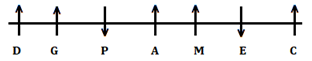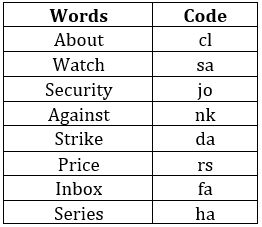Latest Banking jobs   »

# Reasoning Ability Quiz For FCI Phase I 2022- 14th December

Directions (1-5): Study the information carefully and answer the questions given below.
Seven persons sit in a linear row. Some of them face north and some of them face south. A sits second to the right of E. C sits third to the right of A. G sits third to the left of M. Two persons sit between A and D who is not an immediate neighbour of E. M is not immediate neighbor of D. Immediate neighbor of M face opposite direction to each other (If one neighbor face north then other will face south vice versa). G and P sits immediate right to each other. D face same direction as M who face north. E sits immediate left of C.

Q1. How many persons face south direction?
(a) One
(b) Four
(c) Three
(d) Five
(e) None of these

Q2. How many persons sits between P and C?
(a) Three
(b) One
(c) Two
(d) Four
(e) None of these

Q3. Who among person sits second to the right of P?
(a) M
(b) D
(c) P
(d) G
(e) None of these

Q4. Who among the following person lives immediately right of M?
(a) D
(b) E
(c) C
(d) P
(e) None of these

Q5. Four of the following five are alike in certain way based from a group, find the one that does not belong to that group?
(a) P
(b) D
(c) A
(d)G
(e) M

Directions (6-10): Study the following information carefully and answer the questions given below:
In a certain code language:
‘Security watch against about’ is written as ‘cl sa nk jo’,
‘Strike series inbox price’ is written as ‘ha fa rs da’,
‘About price watch Strike’ is written as ‘sa rs cl da’ and
‘Security about inbox Strike’ is written as ‘cl fa jo da’.

Q6. What is the code for ‘about price’ in the given code language?
(a) cl fa
(b) cl rs
(c) da fa
(d) rs da
(e) None of these

Q7. What is the code for ‘series’ in the given code language?
(a) ha
(b) fa
(c) rs
(d) da
(e) None of these

Q8. What is the code for ‘price’ in the given code language?
(a) ha
(b) fa
(c) rs
(d) da
(e) None of these

Q9. What is the code for ‘Strike price’ in the given code language?
(a) ha da
(b) fa rs
(c) rs da
(d) da fa
(e) None of these

Q10. What is the code for ‘watch security’ in the given code language?
(a) ha sa
(b) fa jo
(c) rs sa
(d) sa jo
(e) None of these

Directions (11-15): In these questions, a relationship between different elements is shown in the statements. The statements are followed by two conclusions. Give answer

Q11. Statement: L≤T≤I, M>I<X, P≤T≥B
Conclusion: I. B<X
II. X>M
(a) if only conclusion II is true.
(b) if only conclusion I is true.
(c) if neither conclusion I nor II is true.
(d) if either conclusion I or II is true.
(e) if both conclusions I and II are true.

Q12. Statement: Z<U≤D, A≤U≤M<S, Q>M≤Y<G
Conclusion: I. Z<Y
II. S>Q
(a) if both conclusion I and II are true.
(b) if only conclusion I is true.
(c) if neither conclusion I nor II is true.
(d) if either conclusion I or II is true.
(e) if only conclusion II is true.

Q13. Statement: N≤T≤I, M>B<X, W<T≤L≥B≥K
Conclusion: I. K≤I
II. I<K
(a) if only conclusion II is true.
(b) if either conclusion I or II is true.
(c) if neither conclusion I nor II is true.
(d) if only conclusion I is true.
(e) if both conclusions I and II are true.

Q14. Statement: L≤T≤I, M>I<X, P≤T≥B
Conclusion: I. M≥P
II. X>L
(a) if only conclusion II is true.
(b) if only conclusion I is true.
(c) if neither conclusion I nor II is true.
(d) if either conclusion I or II is true.
(e) if both conclusions I and II are true.

Q15. Statement: Z<U≤D, A≤U≤M<S, Q>M≤Y<G
Conclusion: I. D>S
II. S≤D
(a) if only conclusion II is true.
(b) if either conclusion I or II is true.
(c) if neither conclusion I nor II is true.
(d) if only conclusion I is true.
(e) if both conclusions I and II are true.

Solutions

Solutions (1-5):
Sol.S1. Ans. (e)
S2. Ans. (a)
S3. Ans. (b)
S4. Ans. (b)
S5. Ans. (a)

Solutions (6-10):
Sol.S6. Ans. (b)
S7. Ans. (a)
S8. Ans. (c)
S9. Ans. (c)
S10. Ans. (d)

S11. Ans. (b)
S12. Ans. (b)
S13. Ans. (b)
S14. Ans. (a)
S15. Ans. (c)#### Congratulations!Union Budget 2023-24: Free PDF